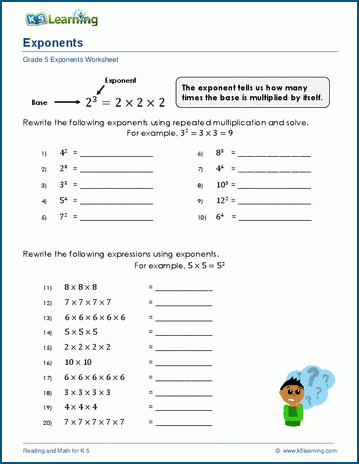# Exponents Worksheets

## Reading and writing exponents worksheets

Our grade 5 exponents worksheets introduce the use of exponents, including the special case of powers of ten.

## Exponents

Introducing exponents 23 = 2 x 2 x 2
Writing exponents 3 x 3 x 3 x 3 =

## Powers of Ten

Powers of Ten 5 x 105 =